# Nickzom Calculator Solves Linear Algebra

Linear Algebra is a matrix based mathematical computation analysis.

According to Wikipedia,

Linear algebra is central to almost all areas of mathematics. For instance, linear algebra is fundamental in modern presentations of geometry, including for defining basic objects such as linesplanes and rotations. Also, functional analysis may be basically viewed as the application of linear algebra to spaces of functions. Linear algebra is also used in most sciences and engineering areas, because it allows modeling many natural phenomena, and efficiently computing with such models. For nonlinear systems, which cannot be modeled with linear algebra, linear algebra is often used as a first-order approximation.

Nickzom Calculator solves 8 major sections of Linear Algebra such as:

• Matrix Arithmetic
• Determinant of a Square Matrix
• Minors and Cofactors of a Square Matrix
• Transpose of a Matrix
• Adjoint of a Square Matrix
• Inverse of a Square Matrix
• Eigenvalues and Eigenvectors of a Square Matrix
• Simultaneous Equation using Inverse Method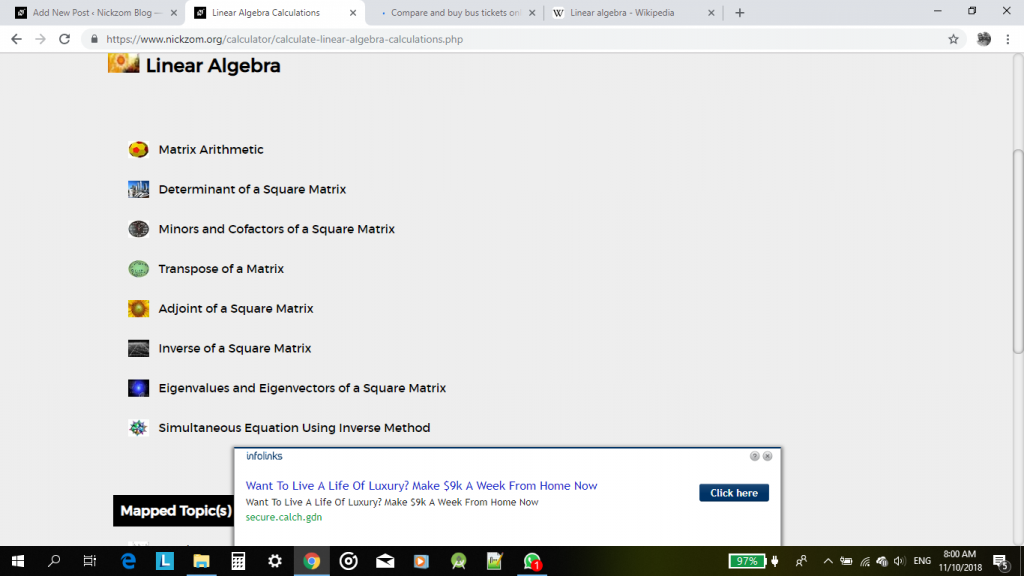Under, Matrix Arithmetic, there are 6 sub sections which are:

• Subtraction of Matrices
• Multiplication of a Matrix by a Scalar
• Multiplication of Two Matrices
• Division of a Matrix by a Square Matrix

For the purpose of this post, I would love for us to find the inverse of a 3 by 3 square matrix.

First you enter the number of rows in the matrix. In this case, it is 3.Next, you enter the matrix values according to rows in this format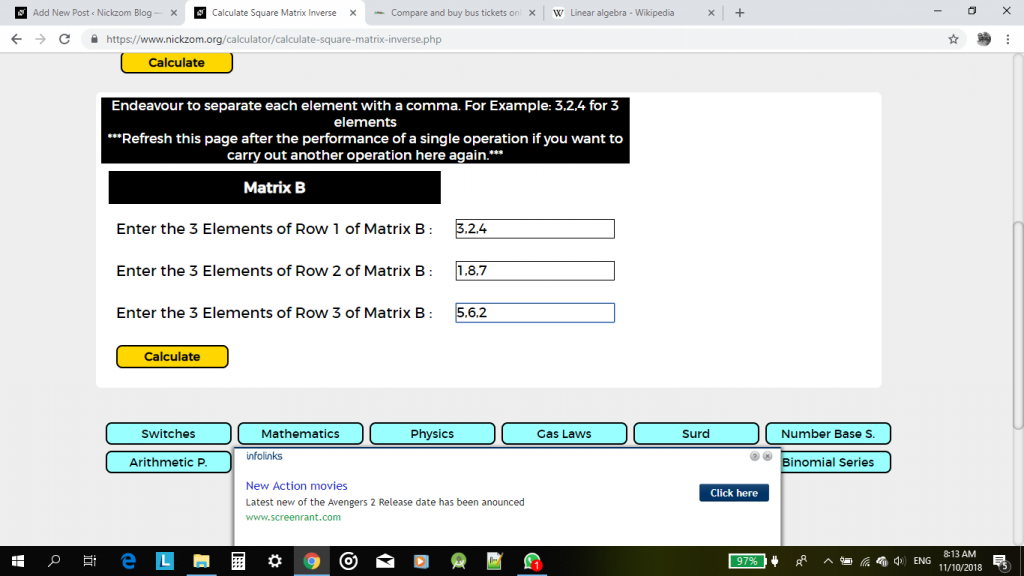Now click on calculate.

Step 1: It provides the determinant of the square matrix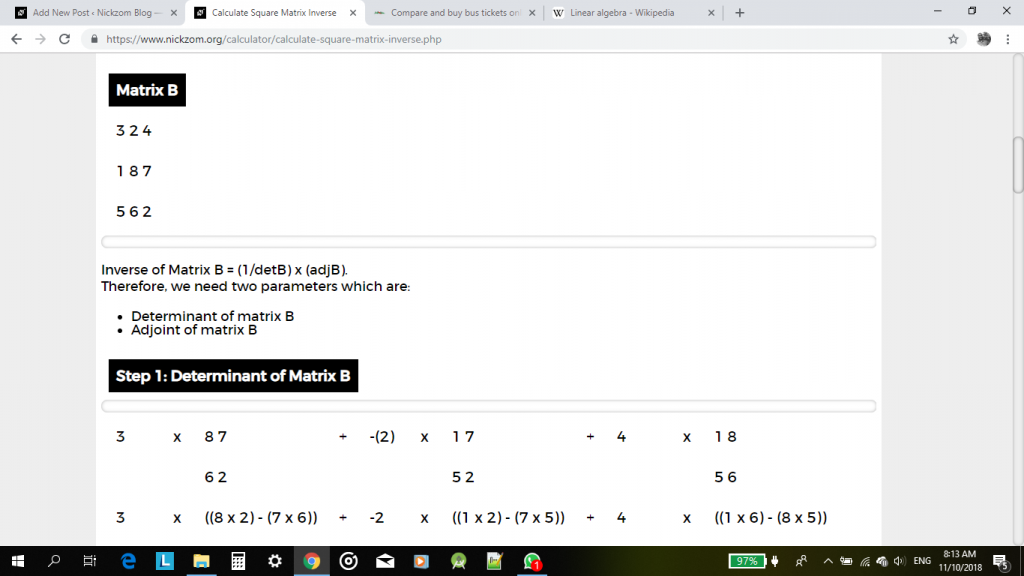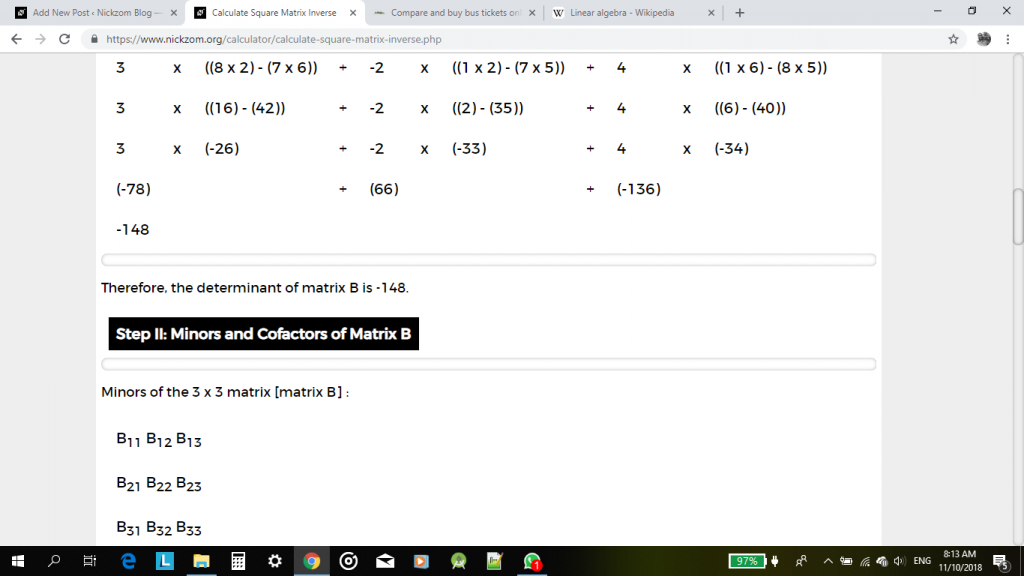Step 2: It determines the minors and cofactors of the matrix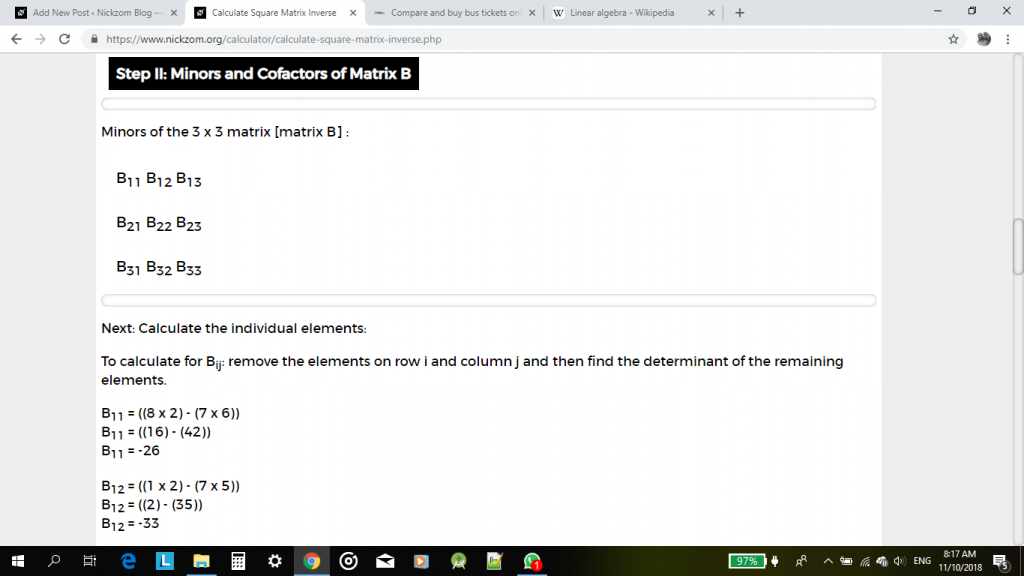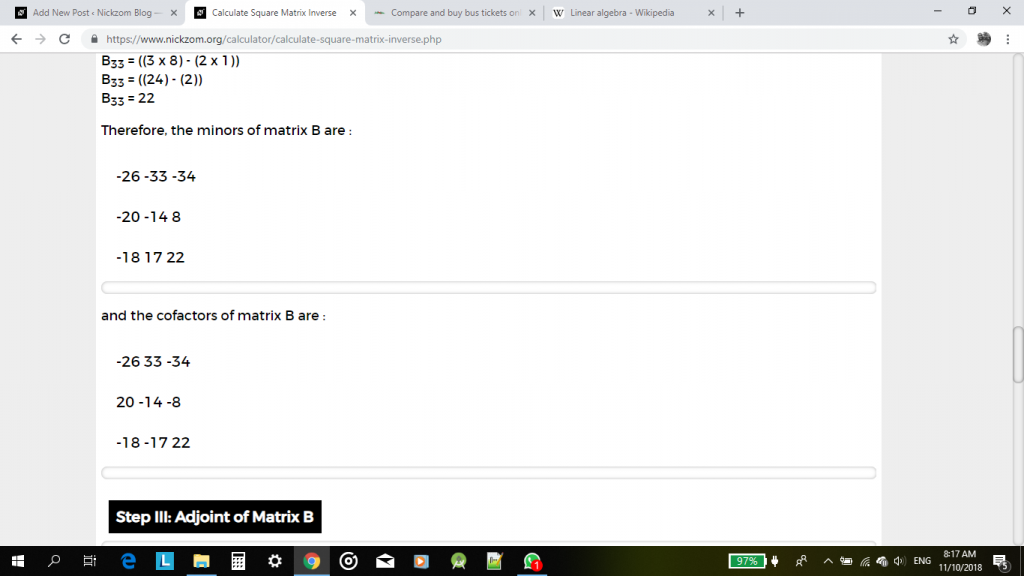Step 3: It determines the adjoint of the matrix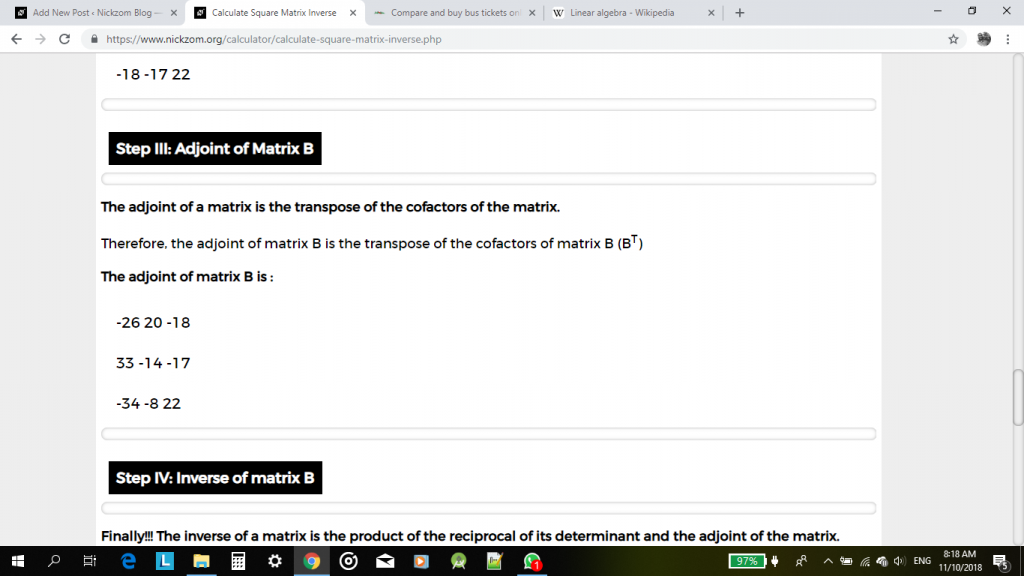Step 4: It finally produces the inverse of the matrix.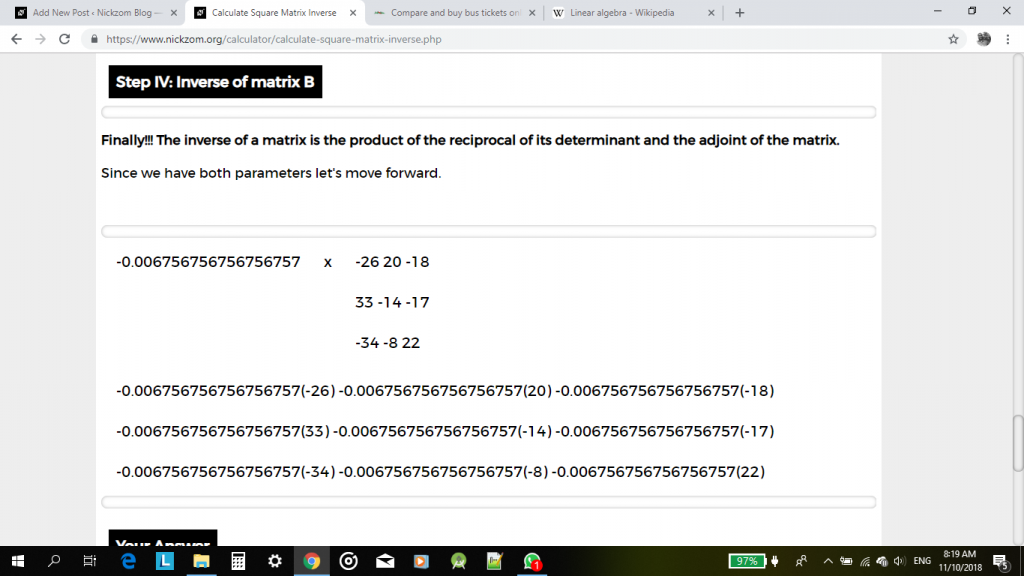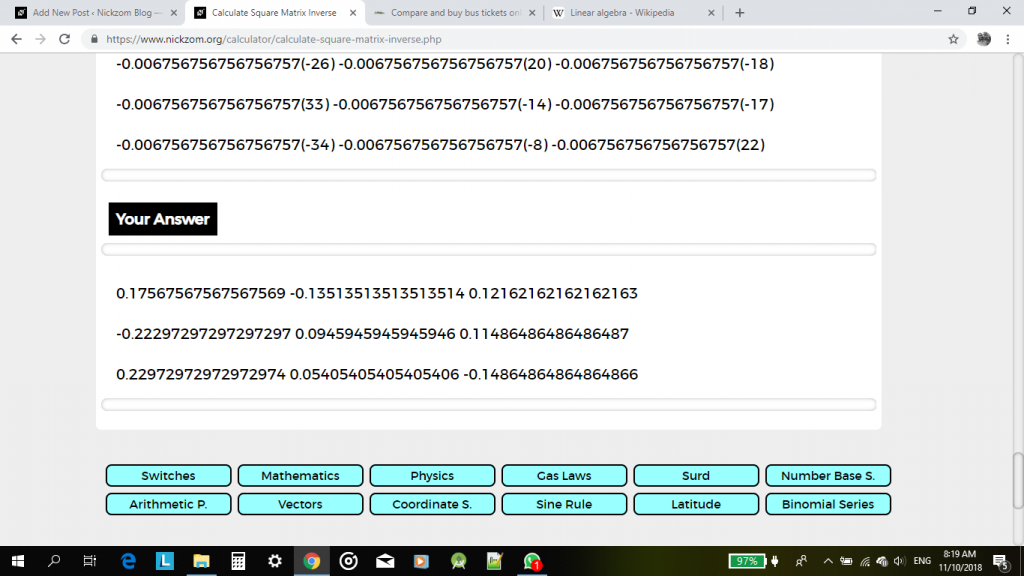Very easily, right?

Nickzom Calculator is simply the best.

One can access Nickzom Calculator via any of these channels: